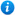* Watermarks do not appear on purchased resources

Preview resource: Click slides to enlarge

# Negative Numbers - Year 6

KS2 Maths Teaching Resources: Negative Numbers - Year 6

Year 6 maths programme of study - Number (number and place value):

• use negative numbers in context, and calculate intervals across 0

In 'Negative Numbers - Year 6', pupils will practise how to use negative numbers in context, and calculate intervals across zero. This KS2 maths resource is ideal to use in a lesson covering the curriculum objectives of the year 6 maths programme of study (Number - number and place value) listed above.

'Negative Numbers - Year 6' is an animated PowerPoint presentation containing:

• A negative numbers recap with link to explanatory video
• Comparing negative numbers using the > and < signs activity and accompanying worksheet
• Ordering negative numbers activity with accompanying worksheet
• Identifying temperatures and calculating the difference between two temperatures activity with accompanying worksheet
• A negative numbers addition and subtraction explanation
• A negative numbers addition and subtraction activity with accompanying worksheet
• Adding and subtracting negative numbers explanation
• Adding and subtracting negative numbers activity with accompanying worksheet
• Printable 'Connect Three' negative numbers game
• links to external negative numbers videos and games
'Negative Numbers - Year 6' is editable so teachers can adapt the resource to meet the needs of each class they teach.

Click on the PowerPoint images opposite to preview 'Negative Numbers - Year 6'.

Our Price : £2.99 / 3 Credits